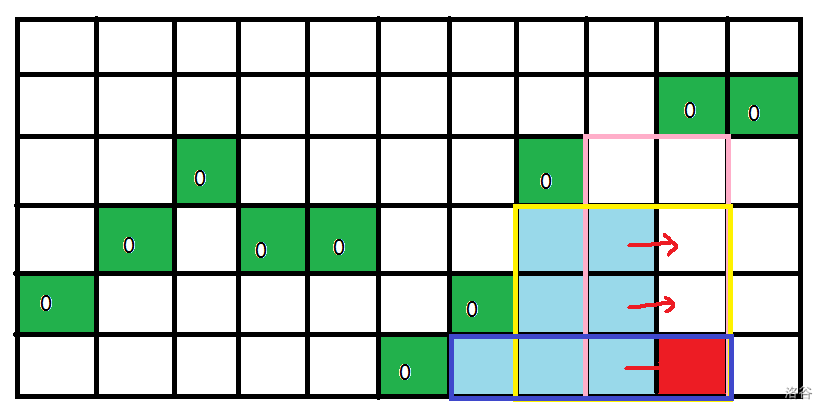01矩阵，求全1的子矩阵数目

# 解析

``````#include<cstdio>
#define int long long
int n,m,area;
int low;//low[j]表示第j列最高的破坏点（0）
int f;//f[i]表示当前计算的这一行，第i列的答案
int s,top;//手写栈
int ans;
signed main(){
scanf("%lld%lld",&n,&m);
for(int i=1;i<=n;i++)
for(int j=1;j<=m;j++)
scanf("%lld",&area[i][j]);
for(int i=1;i<=n;i++)
for(int j=1;j<=m;j++){
if(!area[i][j])low[j]=i;//在这个时候更新最高破坏点
while(top&&low[s[top]]<low[j])top--;
//如果一个选手比你小，还比你强，那我们让他退役...... qvq
ans+=(f[j]=f[s[top]]+(i-low[j])*(j-s[top]));
//使用手写栈，栈空时栈顶元素值为0，而非RE
//其实这一行好多题解都有点难懂，我写的不太一样
//我写的是由s[top]转移而来，而不是从j-1
//可以对着洛谷题解的图理解，但是那张图的矩形颜色跟我想的不一样啊（蓝色区域为什么啊qvq）
//我这里加的是粉色矩形，挺好理解的吧qvq
s[++top]=j;
//我习惯这个时候再入栈，多舒服
}
top=0;//每一行新开一个栈
printf("%lld\n",ans);
}``````

# 附图